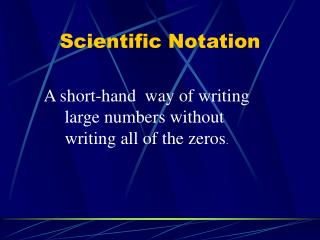DownloadDownload PresentationScientific Notation

Scientific Notation

Télécharger la présentationScientific Notation

- - - - - - - - - - - - - - - - - - - - - - - - - - - E N D - - - - - - - - - - - - - - - - - - - - - - - - - - -
Presentation Transcript

1. Scientific Notation A short-hand way of writing large numbers without writing all of the zeros.

2. The Distance From the Sun to the Earth 93,000,000

3. Step 1 • Move decimal left • Leave only one number in front of decimal 93,000,000 = 9.3000000

4. Step 2 • Drop the zeros after the decimal point • This number is called the COEFFICIENT 9.3000000 = 9.3

5. Step 3 • Multiply your COEFFICIENT by 10…just write the X 10. 9.3 x 10

6. 7 93,000,000 = 9.3 x 10 Step 4 • Count how many places originally you moved decimal • Make that your power of ten

7. The power of ten is 7 because the decimal moved 7 places. 7 93,000,000 = 9.3 x 10

8. 93,000,000 --- Standard Form • 9.3 x 107 --- Scientific Notation

9. 9.85 x 107 -----> 6.41 x 1010 -----> 2.79 x 10-6 -----> 4.2 x 106 -----> Practice Problem Write in scientific notation. Decide the power of ten. • 98,500,000 = 9.85 x 10? • 64,100,000,000 = 6.41 x 10? • 0.00000279 = 2.79 x 10? • 4,200,000 = 4.2 x 10?

10. More Practice Problems On these, decide where the decimal will be moved. • 0.0000681 = ______ x 10-5 • 870,000,000,000 = ______x 1011 • 90,000,000,000 = _____ x 1010 Answers 3) 9 x 1010 • 6.81 x 10-5 2)8.7 x 1011

11. Complete Practice Problems Write in scientific notation. • 50,000 • 0.0000072 • 802,000,000,000 Answers 1) 5 x 104 2) 7.2 x 10-6 3) 8.02 x 1011

12. 3.40000 --- move the decimal ---> Scientific Notation to Standard Form If exponent is positive, move decimal to the RIGHT. If exponent is negative, move decimal to the LEFT! • 3.4 x 105 in scientific notation • 340,000 in standard form

13. 6.27 x 106 9.01 x 10-4 6,270,000 0.000901 Write in Standard Form Move the decimal to the right.